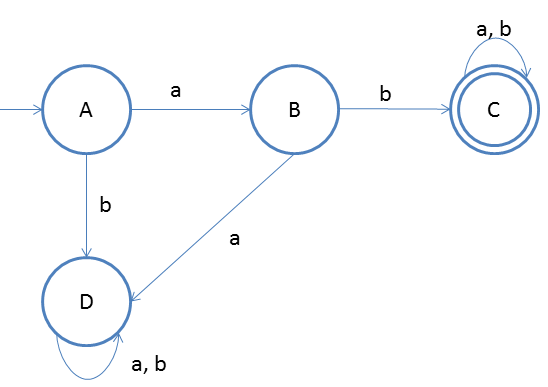# DFA String Examples

We will now discuss about string patterns such as, starting with some combo of symbols, ending with some combo of symbols, etc.

Design a DFA in which set of all strings can be accepted which start with ab.
Given: Input alphabet, Σ={a, b}
Language L ={ab, aba, abaabbb, abba, ….}

Clearly the language is infinite because there is infinite number of strings.The idea behind this approach is very simple, follow the steps below and you will understand.

1. First we will make DFA for accepting the smallest string that is ‘ab’ and after that whatever comes we don’t care as the condition is satisfied.
2. In DFA we have to take care of all the input alphabets at every state.
3. so we have to take care of input symbol ‘b’ on state A, that is we have to reject it cause first ‘a’ should come then ‘b’.
4. So we will make one more state D to take care of ‘b’ from state A
5. On state B if a comes then we have to reject it, so we will direct this input to state D
6. On state D for input a and b we do not care so we will make one self-loop

### Testing

1. Lets take one input string abab
2. First input is a, so from state A we will go to state B
3. Second input is b, so from state B we will go to state C
4. Third input is a, so from state C we will go to state C itself
5. Fourth input is b, so from state C we will go to state C itself(final state)
After end of the string we are at final state so string is accepted.